# Class 9 Maths Chapter 9 Areas of Parallelogram and Triangles MCQs

Class 9 Maths Chapter 9 Areas of Parallelogram and Triangles MCQs are available here online. Students can prepare for the final exams by solving these objective questions to score good marks. The questions here are provided with answers and detailed explanations, as per the CBSE syllabus and NCERT curriculum. Students can solve the chapter-wise MCQs at BYJU’S and also check Important Questions for Class 9 Maths.

## MCQs on Class 9 Maths Chapter 9 Areas of Parallelogram and Triangles

Solve the multiple-choice questions here and choose the correct answer from the given four options.

1) If ABCD is a parallelogram, AE ⊥ DC and CF ⊥ AD. If AB = 10 cm, AE = 6 cm and CF = 5 cm, then AD is equal to:

a. 10cm

B.6cm

c. 12cm

d. 15cm

Explanation: Given,

AB = CD = 10 cm (Opposite sides of a parallelogram)

CF = 5 cm and AE = 6 cm

Now,

Area of parallelogram = Base × Altitude

CD × AE = AD × CF

10 × 6 = AD × 5

2) If E, F, G and H are the mid-points of the sides of a parallelogram ABCD, respectively, then ar (EFGH) is equal to:

a. 1/2 ar(ABCD)

b. ¼ ar(ABCD)

c. 2 ar(ABCD)

d. ar(ABCD)

Explanation: Join H and F as shown in the below figure: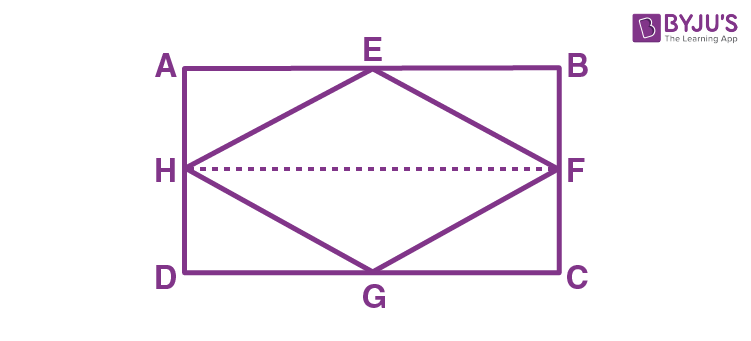AH || BF and and DH || CF

AH = BF and DH = CF (H and F are midpoints)

∴, ABFH and HFCD are parallelograms.

ΔEFH and llgmABFH, both lie on a common base, FH.

∴ area of EFH = ½ area of ABFH — 1

area of GHF = 1/2area of HFCD — 2

Adding eq. 1 and 2 we get;

area of ΔEFH + area of ΔGHF = ½ (area of ABFH + area of HFCD)

ar (EFGH) = ½ ar(ABCD)

3) If P and Q are any two points lying on the sides DC and AD respectively of a parallelogram ABCD, then:

a. ar(APB) > ar(BQC)

b. ar(APB) < ar(BQC)

c. ar(APB) = ar(BQC)

d. None of the above

Explanation: ΔAPB and parallelogram ABCD lie on the same base AB and in-between same parallel AB and DC.

ar(ΔAPB) = ½ ar(parallelogram ABCD) — 1

ar(ΔBQC) = ½ ar(parallelogram ABCD) — 2

From eq. 1 and 2:

ar(ΔAPB) = ar(ΔBQC)

4) If ABCD and EFGH are two parallelograms between same parallel lines and on the same base, then:

a. ar (ABCD) > ar (EFGH)

b. ar (ABCD) < ar (EFGH)

c. ar (ABCD) = ar (EFGH)

d. None of the above

5) A median of a triangle divides it into two

a. Congruent triangles

b. Isosceles triangles

c. Right triangles

d. Equal area triangles

Explanation: Suppose, ABC is a triangle and AD is the median.

AD is the median of ΔABC.

∴ It will divide ΔABC into two triangles of equal area.

∴ ar(ABD) = ar(ACD) — (i)

also,

ED is the median of ΔABC.

∴ ar(EBD) = ar(ECD) — (ii)

Subtracting (ii) from (i),

ar(ABD) – ar(EBD) = ar(ACD) – ar(ECD)

⇒ ar(ABE) = ar(ACE)

6) In a triangle ABC, E is the mid-point of median AD. Then:

a. ar(BED) = 1/4 ar(ABC)

b. ar(BED) = ar(ABC)

c. ar(BED) = 1/2 ar(ABC)

d. ar(BED) = 2 ar(ABC)

Explanation: See the figure below: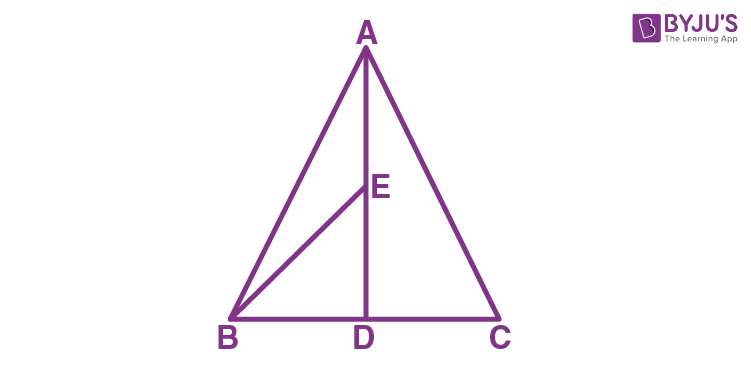ar(BED) = ½ BD.DE

AE = DE (E is the midpoint)

BD = DC (AD is the median on side BC)

DE = ½ AD —- 1

BD = ½ BC —- 2

From eq. 1 and 2, we get;

ar(BED) = (½ ) x (½) BC x (½) AD

ar(BED) = (½) x (½) ar(ABC)

ar(BED) = ¼ ar (ABC)

7) If D and E are points on sides AB and AC respectively of ΔABC such that ar(DBC) = ar(EBC). Then:

a. DE is equal to BC

b. DE is parallel to BC

c. DE is not equal to BC

d. DE is perpendicular to BC

Explanation: ΔDBC and ΔEBC are on the same base BC and also having equal areas.

∴ They will lie between the same parallel lines.

∴ DE || BC.

8) If Diagonals AC and BD of a trapezium ABCD with AB || DC intersect each other at O. Then,

a. ar (AOD) = ar (BOC)

b. ar (AOD) > ar (BOC)

c. ar (AOD) < ar (BOC)

d. None of the above

Explanation: △DAC and △DBC lie on the same base DC and between the same parallels AB and CD.

ar(△DAC) = ar(△DBC)

⇒ ar(△DAC) − ar(△DOC) = ar(△DBC) − ar(△DOC)

⇒ ar(△AOD) = ar(△BOC)

9) If Diagonals AC and BD of a quadrilateral ABCD intersect at O in such a way that ar(△AOD) = ar(△BOC). Then ABCD is a:

a. Parallelogram

b. Rectangle

c. Square

d. Trapezium

Explanation: ar(△AOD) = ar(△BOC)

ar(△AOD) = ar(△BOC)

⇒ ar(△AOD) + ar(△AOB) = ar(△BOC) + ar(△AOB)

Areas of △ADB and △ACB are equal.

Therefore, they must lie between the same parallel lines.

Therefore, AB ∥ CD

Hence, ABCD is a trapezium.

10) If a triangle and a parallelogram are on the same base and between same parallels, then the ratio of the area of the triangle to the area of the parallelogram will be:

a. 1:2

b. 3:2

c. 1:4

d. 1:3

11) The area of the figure formed by joining the mid-points of the adjacent sides of a rhombus with diagonals 12 cm and 16 cm is

(a) 48 cm2

(b) 96 cm2

(c) 64 cm2

(d) 192 cm2

Explanation: Consider the rhombus ABCD as shown in the figure.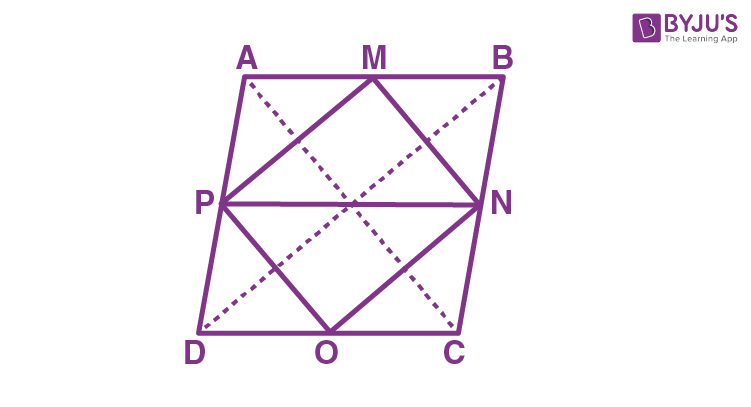Now, join the midpoint of the sides AB, BC, CD, DA of a rhombus ABCD and mark them as M, N, O and P respectively to form a shape MNOP.

Now, join the points P and N to make a line PN. Hence, PN∥AB and PN∥DC

From the above diagram,  parallelogram ABNP and triangle MNOP are on the same base PN and in between the same parallel lines PN and AB.

Now, by using the property, “if a triangle and a parallelogram are on the same base and between the same parallels, the area of the triangle is equal to one-half area of the parallelogram”. Hence, we can write

Area of ΔMNP= (½) Area of ABPN…(1)

Area of ΔPON=(½)Area of PNCD…(2)

Hence, the area of ABCD= (½)×d1×d2

By using (i) and (ii), we get

Area of MNOP = Area of ΔMNP+ Area of ΔPON

=(½)area of ABPN+(½)area of PNDC

=½((½)×12×16) = 48cm2.

12) Parallelogram is a

(a) Pentagon

(c) Heptagon

(d) Octagon

Explanation: Parallelogram is a quadrilateral, as it has four sides.

13) The figure obtained by joining the mid-points of the adjacent sides of a rectangle of sides 8 cm and 6 cm is

(a) a square of area 25 cm2

(b) a rectangle of area 24 cm2

(c) a rhombus of area 24 cm2

(d) a trapezium of area 24 cm2

Explanation: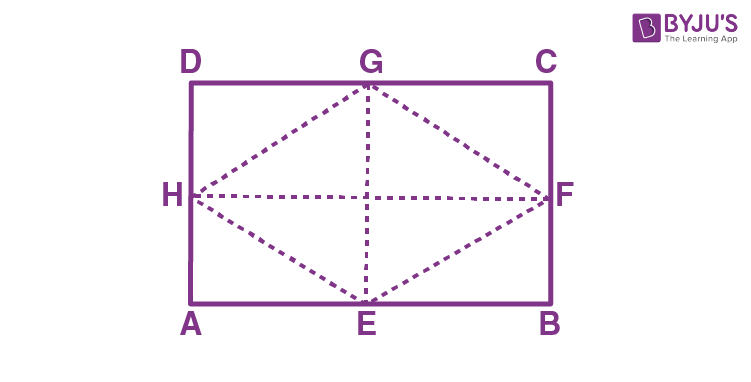Let ABCD be a rectangle, and E, F, G and H are the midpoints of AB, BC, CD and DA respectively.

Hence, the figure obtained by joining the midpoints E, F, G and H is a rhombus.

Now, we need to find the area of the rhombus.

Since, the side lengths of the rectangle are given,

Diagonal of rhombus FH = Side length of the rectangle AB = 8 cm

Diagonal of rhombus EG = Side length of the rectangle AD = 6 cm

Therefore, the area of rhombus = (½)(AB)(AD)

= (½)(8)(6) = 48/2 = 24 cm2.

14) The area of parallelogram ABCD for the following figure is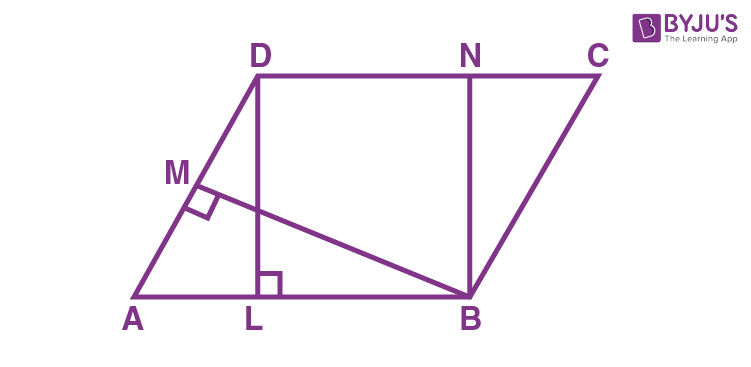(a) BC × BN

(b) AB × BM

(c) DC × DL

Explanation: The area of a parallelogram for the given figure is DC × DL, as the formula for the area of the parallelogram is base×height.

15) Two parallelograms are on equal bases and between the same parallels. The ratio of their areas is

(a) 1: 1

(b) 1: 2

(c) 2: 1

(d) 3: 1

Explanation: We know that the parallelogram on the equal bases and between the same parallels are equal in area. Hence, the ratio of their areas is 1 :1.

16) The mid-point of the sides of a triangle along with any of the vertices as the fourth point make a parallelogram of area equal to

(a) ar (ABC)

(b) (1/2) ar(ABC)

(c) (1/3) ar(ABC)

(d) (1/4) ar(ABC)

Explanation:

Now, consider a triangle ABC as shown in the figure.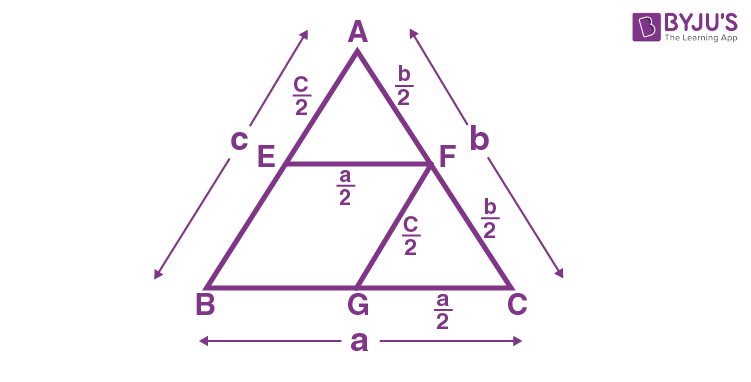Let the area of the triangle be “A”.

By using Heron’s formula

Therefore, the area of triangle AEF = A/4

Area of triangle CFG = A/4

Therefore, the area of parallelogram BEFG = Area of triangle ABC – Area of AEF – Area of CFG

= A – (A/4) – (A/4)

= (4A – A – A)/4

= 2A/4

=(½) A.

Hence, option (B) is the correct answer.

17) The area of a parallelogram with base “b” and height “h” is

(a) b×h square units

(b) b2 square units

(c) h2 square units

(d) b+h square units

Explanation: The area of a parallelogram with base “b” and height “h” is b×h.(i.e) A = base × height square units.

18) ABCD is a quadrilateral whose diagonal AC divides it into two parts, equal in area, then ABCD

(a) is always a rhombus

(b) is a rectangle

(c) is a parallelogram

(d) need not be any of (A), (B) or (C)

Explanation: A quadrilateral ABCD need not be any of rhombus, parallelogram or rectangle. If ABCD is a square, its diagonal AC divides it into two parts and are equal in area.

19) If parallelogram ABCD and rectangle ABEM are of equal area for the given figure, then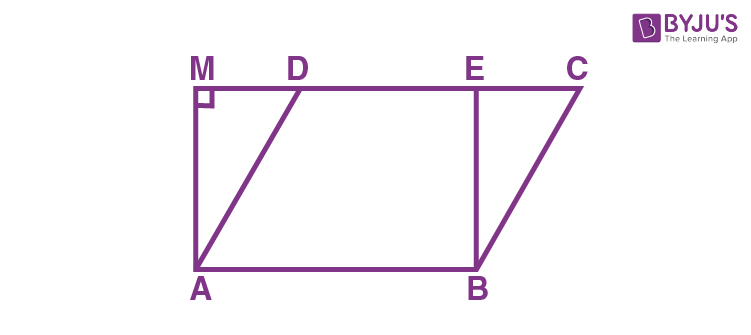(a) Perimeter of ABCD = Perimeter of ABEM

(b) Perimeter of ABCD = (½) Perimeter of ABEM

(c) Perimeter of ABCD < Perimeter of ABEM

(d) Perimeter of ABCD > Perimeter of ABEM

Explanation: Perimeter of parallelogram ABCD is greater than Perimeter of rectangle ABEM

20) Triangles on the same base and between the same parallels are

(a) Greater in area

(b) Equal in area

(c) smaller in area

(d) None of the above